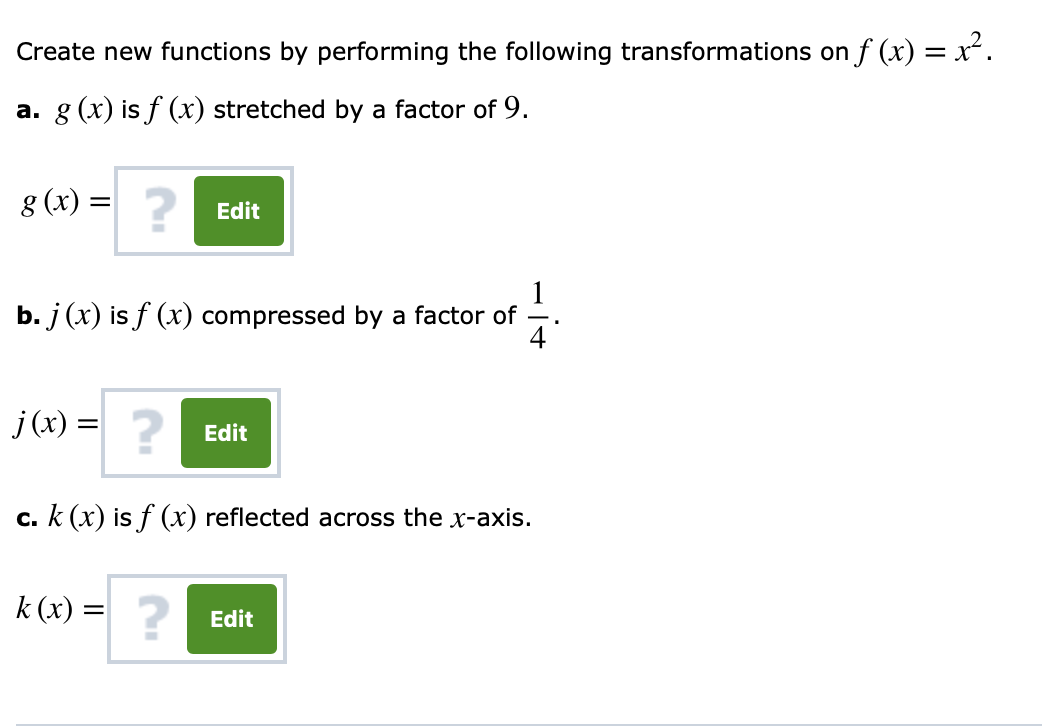# Create new functions by performing the following transformations on f (x)-a. g (x) is f (x) stretched by a factor of 9g (x) =2Editb.j (r) is f (r) compressed by a factor of4JCx)c. k (r) is f (x) reflected across the x-axis.k (x)-2Edit2Edit

Question
1 viewshelp_outlineImage TranscriptioncloseCreate new functions by performing the following transformations on f (x)- a. g (x) is f (x) stretched by a factor of 9 g (x) = 2 Edit b.j (r) is f (r) compressed by a factor of 4 JCx) c. k (r) is f (x) reflected across the x-axis. k (x)- 2 Edit 2 Edit fullscreen
check_circle

Step 1

The given function f(x)=x2

Step 2

Find the new function j(x) .

Step 3

(c) Find the new function ...

### Want to see the full answer?

See Solution

#### Want to see this answer and more?

Solutions are written by subject experts who are available 24/7. Questions are typically answered within 1 hour.*

See Solution
*Response times may vary by subject and question.
Tagged in